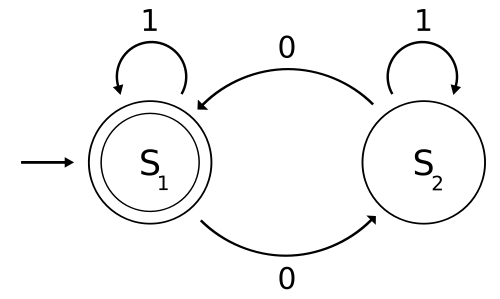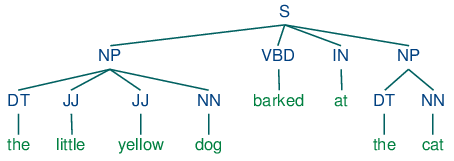# DFA, NFA, Regular expression

NFA 和 DFA 最大的不同在於轉移狀態時，考慮的路徑情況，NFA 可以在不考慮輸入進行轉移，或者在相同情況下可以有很多轉移選擇。 DFA 則必須在一個輸入唯一一個轉移情況下運作。# CFG (Context-free grammar)

A context-free grammar is a formal system that describes a language by specifying how any legal string (i.e., sequence of tokens) can be derived from a start symbol. It consists of a set of productions, each of which states that a given symbol can be replaced by a given sequence of symbols.

• CFG G = (Vt, Vn, S, P)

• Vt: 有限的 terminal 字符集
• Vn: 有限的 nonterminal 字符集
• S: 一開始出發的 start symbol
• P: 轉換的規則 (production)
• 來個例子

LHS (left-hand side) -> RHS (right-hand side)

<E>         -> <Prefix> ( <E> )
<E>         -> V <Tail>
<Prefix>    -> F
<Prefix>    -> l
<Tail>      -> + <E>
<Tail>      -> l


明顯地

• Vt = {(, ), V, F, l, +}
• Vn = {<E>, <Prefix>, <Tail>}
• S = <E>
• P 就是給定那六條規規則
• 名詞
• Sentential form
If S =>* β, β then is said to be sentential form of the CFG
簡單來說，所有從 start symbol 過程中替換出來的句子都稱為 sentential form。
• Handle of a sentential form
The handle of a sentential form is the left-most simple phrase.簡單來說，就是將一個 nonterminal 根據 production 轉換成 RHS (right-hand side) 的一個步驟。
• 什麼是 CFG (上下文無關語法)？
簡單來說，還真的是上下文無關，例如 S -> AB 可以見到 S 轉換時，不會因為前文或後文是什麼內容而影響，而在 Context-sensitive grammar (上下文敏感) 可以見到 aSb -> AB 會因為前面跟後面所受到的影響。

## First Set

• First(A)
• The set of all the terminal symbols that can begin a sentential form derivable from A. A =>* aB
First(A) = {
a in Vt | A =>* aB, and
Λ in Vt | A =>* Λ
}

• 簡單地說，從這個 nonterminal 開始，第一個不能被替換的 terminal 就會在 First set 中。
• 計算 First(A) 前，必須先建出這個 nonterminal 有沒有可能推出 Λ，即 A =>* Λ
這邊相當有意思，使用迭代更新，如果發現 B =>* ΛC =>* Λ 則對於 Production A => BC 來說，可以得到 A =>* Λ 就這麼依序推出所有情況，時間複雜度應該在 O(n^2)
• 計算 First(A) 時，掃描規則 LHS => RHS，找到 First(RHS) 並且 First(LHS).insert(First(RHS))，依樣使用迭代更新推出所有情況，時間複雜度應該在 O(n^2)

• Follow(A)
• A is any nonterminal
• Follow(A) is the set of terminals that may follow A in some sentential form. S =>* ... Aabc ...follow set。
Follow(A) = {
a in Vt | S =>* ... Aa ..., and
Λ in Vt | S =>* aA
}

• 請切記，一定要從 start symbol 開始替換的所有句子，而在這個 nonterminal 隨後可能跟著的 termainl 就會屬於它的。
• 計算 Follow(A) 前，請先把 First set 都算完，接著也是用迭代更新，掃描規則 A -> a B b
對於

if Λ in First(X1 X2 … Xm)
Predict(A -> X1 X2 … Xm).insert(First(X1 X2 … Xm) - Λ);
Predict(A -> X1 X2 … Xm).insert(Follow(A));
else
Predict(A -> X1 X2 … Xm).insert(First(X1 X2 … Xm));

S->aSe
S->B
B->bBe
B->C
C->cCe
C->d

A->BaAb
A->cC
B->d
B->l
C->eA
C->l

A->B
B->bBd
B->C
C->cCd
C->l

E->TX
X->+E
X->#
T->(E)
T->intY
Y->*T
Y->#

E->P(E)
E->vT
P->f
P->l
T->+E
T->l

S->aSe
S->B
B->bBe
B->C
C->cCe
C->d

S->ABc
A->a
A->l
B->b
B->l

B:b,c,d
C:c,d
S:a,b,c,d
a:a
b:b
c:c
d:d
e:e
A:a,c,d
B:d,l
C:e,l
a:a
b:b
c:c
d:d
e:e
A:a,b,c,l
B:b,c,l
C:c,l
a:a
b:b
c:c
d:d

#:#
(:(
):)
:
+:+
E:(,i
T:(,i
X:#,+
Y:#,*
i:i
n:n
t:t
(:(
):)
+:+
E:(,f,v
P:f,l
T:+,l
f:f
v:v
B:b,c,d
C:c,d
S:a,b,c,d
a:a
b:b
c:c
d:d
e:e
A:a,l
B:b,l
S:a,b,c
a:a
b:b
c:c

-> begin end
->
->
-> l
-> ID := ;
-> write ( ) ;
-> ID
-> , ID
-> l
->
-> ,
-> l
->
->
-> l
-> ( )
-> ID
-> INTLIT
-> +
-> -
-> $begin ID := ID - INTLIT + ID ; end$

+—————-+—– First set —–+
|$| {$ }
|( | { ( }
|) | { ) }
|+ | { + }
|, | { , }
|- | { - }
|:= | { := }
|; | { ; }
| | { + - }
| | { ( ID INTLIT }
| | { , l }
| | { ( ID INTLIT }
| | { ID }
| | { , l }
| | { ( ID INTLIT }
| | { + - l }
| | { begin }
| | { ID read write }
|| { ID read write }
|| { ID l read write }
| | { begin }
|ID | { ID }
|INTLIT | { INTLIT }
|begin | { begin }
|end | { end }
|write | { write }
+—————-+———————+

| | { ( ID INTLIT }
| | { ) }
| | { ) }
| | { ) , ; }
| | { ) }
| | { ) }
| | { ) + , - ; }
| | { ) , ; }
| | { $} | | { ID end read write } || { end } || { end } | | { l } +—————-+———————+ +—————-+—– LL(1) table —–+ | |$| (| )| +| ,| -| :=| ;| ID|INTLIT| begin| end| read| write|
| | | | | 20| | 21| | | | | | | | |
| | | 11| | | | | | | 11| 11| | | | |
| | | | 13| | 12| | | | | | | | | |
| | | 14| | | | | | | 14| 14| | | | |
| | | | | | | | | | 8| | | | | |
| | | | 10| | 9| | | | | | | | | |
| | | 17| | | | | | | 18| 19| | | | |
| | | | 16| 15| 16| 15| | 16| | | | | | |
| | | | | | | | | | | | 1| | | |
| | | | | | | | | | 5| | | | 6| 7|
|| | | | | | | | | 2| | | | 2| 2|
|| | | | | | | | | 3| | | 4| 3| 3|
| | | | | | | | | | | | 22| | | |
+—————-+———————+

Process syntax accept



## 代碼

2014/6/1 修正 parsing 時的 lambda 問題。